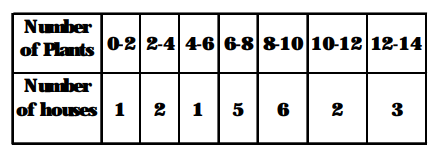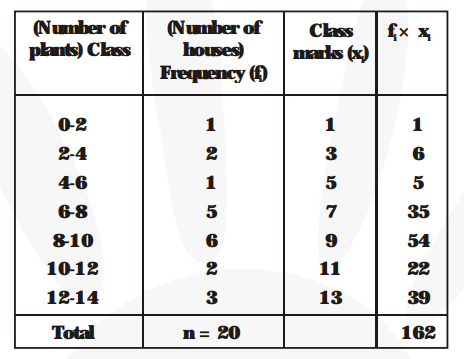# A survery was conducted by a group of students as a part of their environment awareness programme,Question:

A survery was conducted by a group of students as a part of their environment awareness programme, in which they collected the following data regarding the number of plants in 20 houses in a locality. Find the mean number of plants per house.Which method did you use for finding the mean, and why?

Solution:

Let us find mean of the data by direct method because the figures are small.We have, $\mathrm{n}=\Sigma \mathrm{f}_{\mathrm{i}}=20$ and $\Sigma \mathrm{f}_{\mathrm{i}} \mathrm{x}_{\mathrm{i}}=162$.

Then mean of the data is

$\overline{\mathbf{x}}=\frac{\mathbf{1}}{\mathbf{n}} \times \Sigma \mathrm{f}_{\mathrm{i}} \mathrm{x}_{\mathrm{i}}=\frac{\mathbf{1}}{\mathbf{2 0}} \times 162=8.1$

Hence, the required mean of the data is $8.1$ plants.